## Fixed beam calculator

This tool calculates the static response of beams, with both their ends fixed, under various loading scenarios. The tool calculates and plots diagrams for these quantities:

• reactions
• bending moments
• transverse shear forces
• deflections
• slopes

Please take in mind that the assumptions of Euler-Bernoulli beam theory are adopted, the material is elastic and the cross section is constant over the entire beam span (prismatic beam).

Units:
 1 2 3 4 Structure L = mcmmmydftin Optional properties, required only for deflection/slope results: E = PakPaMPaGPapsiksiMpsi I = m^4cm^4mm^4ft^4in^4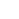Calculate the moment of inertia of various beam cross-sections, using our dedicated calculators.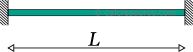ADVERTISEMENT
 1 2 3 4 Results: Reactions: RA = kNNkgtlbfkip RB = kNNkgtlbfkip MA = kNmNmkg.mt.mlbf.ftlbf.inkip.ftkip.in MB = kNmNmkg.mt.mlbf.ftlbf.inkip.ftkip.in Bending Moment: Mu = kNmNmkg.mt.mlbf.ftlbf.inkip.ftkip.in xm = mcmmmydftin Transverse Shear Force: Vu = kNNkgtlbfkip xv = mcmmmydftin Deflection: du = mcmmmydftin xd = mcmmmydftin Slopes: θA = degradmrad θB = degradmrad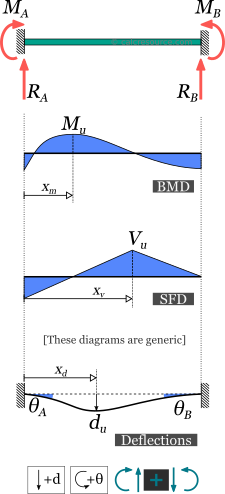1 2 3 4 Request results at a specific point: x = mcmmmydftin M(x) = kNmNmkg.mt.mlbf.ftlbf.inkip.ftkip.in V(x) = kNNkgtlbfkip d(x) = mcmmmydftin θ(x) = degradmrad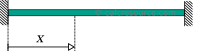## Theoretical background

### Introduction

The fixed beam (also called clamped beam) is one of the most simple structures. It features only two supports, both of them fixed ones. Fixed supports inhibit all movement, including vertical or horizontal displacements as well as rotations. Restraining rotations results in zero slope at the two ends, as illustrated in the following figure.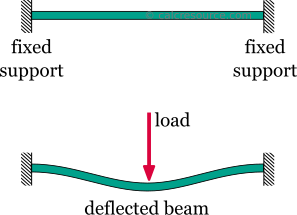The fixed beam features two fixed supports, one at each end

The fixed beam features more supports than required to be statically sound. Indeed the second fixed support could be removed entirely, turning the structure to a cantilever beam, which is still a sound load bearing structure. In other words the fixed beam offers redundancy in terms of supports. To the contrary, a structure without redundancy, would turn to a mechanism, if any of its supports were removed. In this situation the structure could move without restriction in one or more directions. The structures that offer no redundancy, are called critical or determinant structures. If a local failure occurs to these, they would collapse. To the contrary, a structure that features more supports than required to restrict its free movements is called redundant or indeterminate structure. Redundant structures can tolerate one or more local failures before they become mechanisms. The fixed beam is an indeterminate (or redundant) structure.

#### Assumptions

Typically, when performing a static analysis of a load bearing structure, the internal forces and moments, as well as the deflections must be calculated. When the structure is 2-dimensional, and the imposed loads are exercised in the same 2D plane, there are just three resultant actions of interest:

1. the axial forces ,
2. the transverse shear forces , and the
3. bending moments .

For a fixed beam, that is loaded by transverse loads only (so that their direction is perpendicular to the beam longitudinal axis), the axial force is always zero, provided the deflections remain small. Therefore axial forces can be commonly neglected.

The response resultants and deflections presented in this page are calculated taking into account the following assumptions:

• The material is homogeneous and isotropic (in other words its characteristics are the same in ever point and towards any direction)
• The material is linear elastic
• The loads are applied in a static manner (they do not change with time)
• The cross section is the same throughout the beam length
• The deflections are small
• Every cross-section that initially is plane and also normal to the longitudinal axis, remains plane and and normal to the deflected axis too. This is the case when the cross-section height is quite smaller than the beam length (10 times or more) and also the cross-section is not multi layered (not a sandwich type section).

The last two assumptions satisfy the kinematic requirements for the Euler-Bernoulli beam theory and are adopted here too.

#### Sign convention

A sign convention is necessary for the calculation of the internal forces and moments, , and their physical interpretation, at any section cut throughout the beam. The following rules are adopted here:

1. The axial force, , is considered positive when it causes tension to the part
2. The shear force, , is positive when it causes a clock-wise rotation of the part.
3. The bending moment, , is positive when it causes tension to the lower fiber of the beam and compression to the top fiber.

These rules, though not mandatory, are rather universal. A different set of rules, if followed consistently would also produce the same physical results.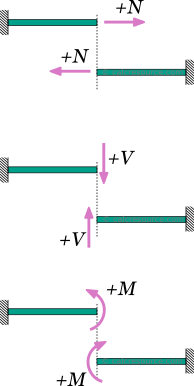Positive sign convention for internal axial force, N, shear force, V, and bending moment, M

#### Symbols

• : the material modulus of elasticity (Young's modulus)
• : the moment of inertia of the cross-section around the elastic neutral axis of bending
• : the total beam span
• : support force reaction
• : deflection
• : bending moment and support moment
• : transverse shear force
• : slope
• : total force of a distributed load

### Fixed beam with uniform distributed load

In this case, the load is distributed uniformly, over the entire beam span, having constant magnitude and direction. Its dimensions are force per length. The total amount of force applied to the beam is , where the span length. In some cases the total force may be given rather than the force per length .

The following table presents the formulas describing the static response of a fixed beam, with both ends fixed, under a uniform distributed load .

### Fixed beam with point force in the middle

In this case, the force is concentrated in a single point, located in the middle of the beam. In practical terms however, the force could be exercised over a limited length. In the close vicinity of the force application, stress concentrations are expected and as result the response predicted by the classical beam theory is maybe inaccurate. This is only a local phenomenon however. As we move away from the force location, the results become valid, by virtue of the Saint-Venant principle, provided the loaded area remains substantially smaller than the total beam length.

The following table presents the formulas describing the static response of a fixed beam, with both ends fixed, under a concentrated point force , imposed in the middle.

### Fixed beam with point force at a random position

In this case, the force is concentrated in a single point, anywhere across the beam span. In practical terms however, the force could be exercised on a small area rather than an ideal point. In order to consider the force as concentrated, though, the dimensions of the loading area should be considerably smaller than the total beam length. In the close vicinity of the force, stress concentrations are expected and as result the response predicted by the classical beam theory maybe inaccurate. This is only a local phenomenon however. As we move away from the force location, the discrepancy of the results becomes negligible.

The following table presents the formulas describing the static response of a fixed beam, with both ends fixed, under a concentrated point force , imposed at a random distance from the left end.

### Fixed beam with point moment

In this case, a moment is imposed in a single point of the beam, anywhere across the beam span. In practical terms, it could be a force couple, or a member in torsion, connected out of plane and perpendicular to the beam, as illustrated in the following figure.

Practically, the moment cannot be exercised on an ideally infinitesimal point. Instead, a small length of the beam, would be subject to the imposed moment action. In the close vicinity the loading area, the predicted results through the classical beam theory are expected to be inaccurate (due to stress concentrations and other localized effects). However, as we move away, the predicted results become perfectly valid, as stated by the Saint-Venant principle, provided the loading area remains substantially smaller than the total beam length.

The following table presents the formulas describing the static response of a fixed beam, with both ends fixed, under a concentrated point moment , imposed at a distance from the left end.

### Fixed beam with triangular load

In this case, the load is distributed throughout the entire beam span, however, its magnitude is not constant. Instead, it is varying linearly, starting from zero at the left fixed end, gradually increasing, up to its peak value , at the right fixed end. The dimensions of are force per length. The total amount of force applied to the beam is , where the span length. In some cases the total force may be given rather than the peak force per length, .The orientation of the triangular load is important for the use of the table! The formulas have been prepared for the case of an ascending load (left-to-right), as shown in the schematic. For a descending load you may mirror the beam, so that its left end (point A) is the least loaded one and consequently, the x axis and related results should be mirrored too.

The following table presents the formulas describing the static response of a fixed beam, with both ends fixed, under a linearly varying (triangular) distributed load, ascending from the left to the right.

### Fixed beam with trapezoidal load

In this case, the load is distributed over the entire beam span, with linearly varying magnitude, starting from at the left fixed end, up to at the right fixed end. The dimensions of and are force per length. The total amount of force applied to the beam is: , where the beam length.The values of and can be freely assigned. It is not mandatory for the former to be smaller than the latter. They may take even negative values (one or both of them).

The following table presents the formulas describing the static response of a fixed beam, with both ends fixed, under a varying distributed load, of trapezoidal form.

### Fixed beam with slab-type trapezoidal load distribution

This load distribution is common for beams in the perimeter of a slab. The shape of the distributed load is trapezoidal, as illustrated in the following figure. The maximum load magnitude is , occurring at the interior of the beam, while at the two sides the load is linearly varying, decreasing to zero at the two ends. The dimensions of are force per length. The total amount of force applied to the beam is: , where the beam length and , the left and right side lengths, respectively, where the load distribution is varying (triangular).

The following table presents the formulas describing the static response of a fixed beam, with both ends fixed, under a trapezoidal load distribution, as depicted in the schematic.

### Fixed beam with partially distributed uniform load

In this case the load is distributed uniformly to only a part of the beam span, having a constant magnitude . The remaining span remains free of any load. The dimensions of are force per length. The total amount of force applied to the beam therefore is: , where is the span length and , are the left and right side lengths, respectively, where no load is imposed.

The following table presents the formulas describing the static response of the fixed beam, with both ends fixed, under a partially distributed uniform load.

### Fixed beam with partially distributed trapezoidal load

In this case, the load is distributed to only a part of the beam length, while the remaining length remains free of any load. The distribution is varying linearly in magnitude from , towards the left side, up to , towards the right side. The dimensions of and are force per length. The total amount of force applied to the beam is therefore: , where is the span length and , are the unloaded lengths at the left and right side of the beam respectively.The values of and can be freely assigned. It is not mandatory for the former to be smaller than the latter. They may take even negative values (one or both of them).This is the most generic case. The formulas for partially distributed uniform and triangular loads can be derived by appropriately setting the values of and . Furthermore, the respective cases for fully loaded span, can be derived by setting and to zero.

The following table presents the formulas describing the static response of the fixed beam, with both ends fixed, under a partially distributed trapezoidal load.

### Related articles

Simply supported beam calculator
Cantilever beam calculator
Fixed-pinned beam calculator
Simply supported beam diagrams
Moments of Inertia Table
All Moment of Inertia tools
All calculators on Statics